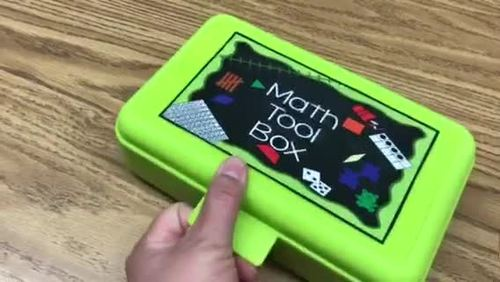# Math Tool Box by KLSubject
Resource Type
File Type

Zip

(2 MB|23 pages)
Standards
• Product Description
• Standards
Kinder League is here to save the day! We have created a Math Tool Box kit to help your students organize their math tools and have them readily accessible. In this download you will get...
4 different color covers
Numbers grids for numbers 1-10, 1,20, 1-30, and 1-50 and a blank to have your students practice number formation and order.
Number chart to 100 (blank)
Number lines 0-10 and 0-20
Ten frame
Five frame
Tens and Ones mat
Number bond
Just laminate and place them in a pencil box.
For any number from 1 to 9, find the number that makes 10 when added to the given number, e.g., by using objects or drawings, and record the answer with a drawing or equation.
Decompose numbers less than or equal to 10 into pairs in more than one way, e.g., by using objects or drawings, and record each decomposition by a drawing or equation (e.g., 5 = 2 + 3 and 5 = 4 + 1).
Compose and decompose numbers from 11 to 19 into ten ones and some further ones, e.g., by using objects or drawings, and record each composition or decomposition by a drawing or equation (e.g., 18 = 10 + 8); understand that these numbers are composed of ten ones and one, two, three, four, five, six, seven, eight, or nine ones.
Count to answer “how many?” questions about as many as 20 things arranged in a line, a rectangular array, or a circle, or as many as 10 things in a scattered configuration; given a number from 1-20, count out that many objects.
Understand that each successive number name refers to a quantity that is one larger.
Total Pages
23 pages
N/A
Teaching Duration
Lifelong tool
Report this Resource to TpT
Reported resources will be reviewed by our team. Report this resource to let us know if this resource violates TpT’s content guidelines.